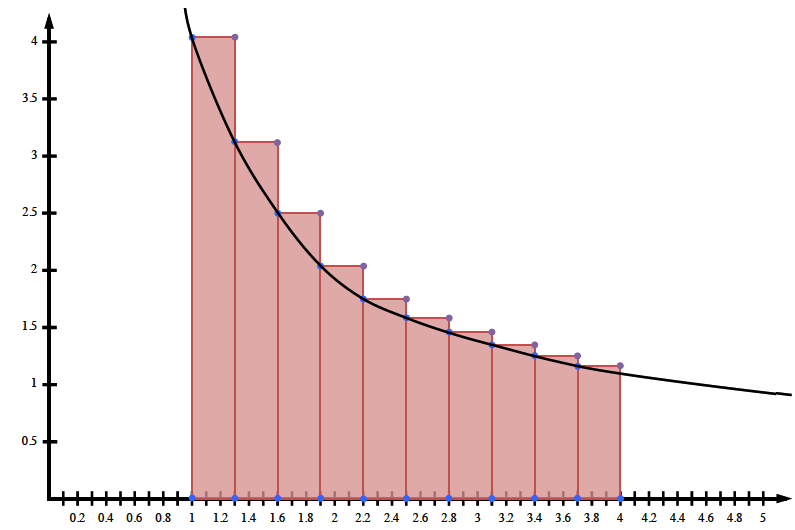### Home > PC > Chapter 7 > Lesson 7.1.2 > Problem7-31

7-31.

Write the sum for estimating $A(\frac { 4 } { x }, 1 ≤ x ≤ 4)$ using $10$ left-endpoint rectangles. Determine if the estimate is an upper bound or lower bound. Justify your answer with a sketch of the curve showing a typical rectangle.

$1. \text{ width}=\frac{\text{length of interval}}{\text{# of rectangles}}$

2. height $= f\left(x\right)$

3. inside function $= \left(\text{width}\right)\left(x + \text{start}\right)$

$\text{Width}\displaystyle \sum_{k =\text{ start}-1}^{\text{end}}\frac{4}{(\text{(width)}(x+\text{start}))}$